Spectral sequences on one blackboard

Spectral sequences are very often referred to as the most forbidding part of learning homological algebra or algebraic topology. The standard presentation is an absolute sea of indices, connecting homomorphisms, kernels and images, filtrations and associated gradeds. They appear only when the lecturer thinks that there’s no way around them, and frequently the lecturer has already made a couple of arguments in the “style” of spectral sequences without actually using those words in some unhappy effort to familiarize the student with the sea of indicies that’s about to come.

But I’m here to tell you that spectral sequences don’t have to be this way! Spectral sequences fit on one board, using one picture, with one index, with obvious motivation, and with minimal misery. This presentation is of course not at all new. It’s still worth saying again in public, on record.

To begin, the key property of a homology functor is that it sends short exact sequences (or cofiber sequences or distinguished triangles or whatever your favorite phrasing and context are) to long exact sequences of homology groups. This is useful in what are now considered very standard ways: objects can be built up out of smaller ones through extensions of these types, and then the homology of the new object is related to the homologies of the new ones.

But, it is rarely the case that objects are given to us in one-step constructions! It is commonly the case that we have a whole filtration: a sequence of subobjects $F_i$, each one stacked in the next, like so: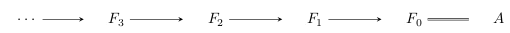Then for each of these inclusions $F_{i+1} \to F_i$, we can build a quotient / cokernel / cofiber object, like this: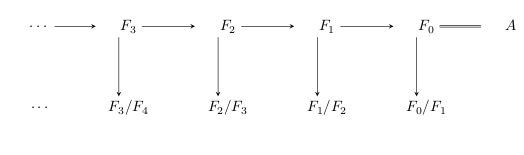Each of these angled pair of arrows is a cofiber sequence, so when we apply homology to this entire diagram, we get going-around maps from the snake lemma: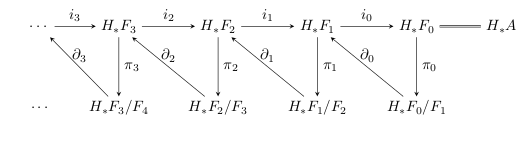These bottom groups are called the first page of the spectral sequence, notated $E^1_{p, q} = H_p F_q / F_{q+1}$ (though this grading varies by author and application). The spectral sequence is going to be an object which interrelates all these long exact sequences.

This diagram as drawn is no longer commutative, so you’ll have to keep aware of that. Instead, each of these triangles is exact in the usual sense that at each node, the image of one map is equal to the kernel of the next. Now, keep in mind our goal: we’re supposed to be reconstructing $H_* A$ from these filtration quotients $H_* F_i / F_{i+1}$. There are two clear problems with looking at the homology of the filtration quotients:

• Maybe we have a cycle that ought to be the boundary of something, but we’re missing that boundary class in this filtration level, or
• Maybe we have an extra cycle coming from collapsing the lower filtration quotient, where there ought to be an obstruction to building the cycle.

These two things are kept track of by arrows that we’ve actually already drawn. Namely, if we choose a class $x \in H_* F_i / F_{i+1}$ that ‘deserves’ to be the preimage of a boundary for some cycle in $H_* F_{i+1} / F_{i+2}$, then the composition $\pi_{i+1} \circ \partial_i$ will spit out that class when applied to $x$. So, we define a sequence of maps $d_1 = \pi_{*+1} \circ \partial_*: H_* F_i / F_{i+1} \to F_{i+1} / F_{i+2}$.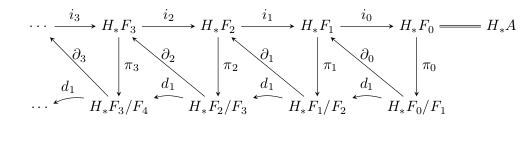These maps in fact form a chain complex – namely, $d_1 \circ d_1 = \pi_{*+2} \circ \partial_{*+1} \circ \pi_{*+1} \circ \partial_*$, and in the middle of this composition we have $\partial_{*+1} \circ \pi_{*+1} = 0$, since they’re two edges of the same exact triangle. So, it makes sense to take homology with respect to these maps, doing which will begin to sort out this business with extra cycles lying around.

So, suppose we do that. The resulting groups $E^2_{p, q}$ are called the second page of the spectral sequence – but then what? Well, what’s left in any filtration degree now is a subquotient of what we started with: we selected only the cycles which did not deserve to be called boundaries one level up and which were not themselves bounded by things one level down — so, in particular, for a class $y$ in these new groups, we have $d_1 y = 0$. So, what does that mean? The map $d_1$ is defined by $\pi_{*+1} \circ \partial_*$, and so we have that $\partial_* y$ is in the kernel of $\pi_{*+1}$. This, in turn, means that $\partial_* y$ has a well-defined preimage $i^{-1}_{*+1} \partial_* y$, which we can again push down to $\pi_{*+2} i^{-1}_{*+1} \partial_* y = d_2$. These are sketched as the dashed maps below: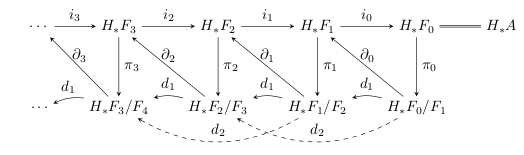Once again, these maps check whether $y$ ought to be involved with the boundary classes in a higher filtration degree – this time two levels up. Once again, the maps $d_2$ form a chain complex and once again taking their homology helps sort out where boundaries belong and don’t belong. This process continues forever, on to higher and higher order pages.

It’s believable, on the face of it, that these groups ‘limit’ to something meaningful with respect to the homology $H_* A$ (called the abutment), but there are fairly serious technical conditions here governing what actually happens (for instance, Boardman wrote a famous 40-ish page paper about them titled Conditionally Convergent Spectral Sequences). The most basic condition to request is that for each homological degree $q$, there is a filtration degree $p$ such that for $p' > p$, $H_q F_{p'} = 0$. This forces these groups to stabilize because it forces us to eventually run out of differentials for each group in the spectral sequence. Then, provided these limiting groups make sense, what’s left over on the $\infty$-page corresponds to filtration quotients of the homology $H_* A$ – so we’ve traded the homology of the filtration for the filtration of the homology. This new problem of stitching the groups together through extensions to recover $H_* A$ is nontrivial but at least tractable – it’s an algebraic question about homology groups rather than a question about whatever source objects we started with.

This is frequently satisfied. For instance, let $X$ be a CW-complex of dimension $n$, and let the filtration $F_k = X^{(n-k)}$ be the skeletal filtration. Then $F_k / F_{k+1}$ is a wedge of spheres, whose singular homology is easily understandable, and the information in the associated spectral sequence is concentrated in such a way that only the differential $d_1$ could possibly be nonzero; all the others vanish for degree reasons. These differentials $d_1$ actually compute the cellular homology of the space, and again the degrees of the nonvanishing groups are stratified in such a way that there are no nontrivial extension problems either. This spectral sequence is said to collapse at the second page, and this proves that cellular homology computes singular homology, just like that. Similarly, we could have used an ascending filtration rather than a descending one, which would remove the dimension restriction on our CW complexes, or we could have used cohomology instead of homology to get a dual statement. More interestingly, we didn’t have to use singular homology at all – we can replace this with any extraordinary homology theory to produce the Atiyah-Hirzebruch spectral sequence, beginning with cellular homology with coefficients in the coefficient ring of some bizarre homology theory and converging to the extraordinary homology of the space itself. Or, starting with a fiber bundle over a CW-complex, we can filter the total space by preimages of the skeleta in the base, which (though it takes some work to show this) produces the Serre spectral sequence.

Here’s a more radical example of how you can easily roll your own spectral sequences with this setup: one model for the classifying space $BG$ uses a simplicial version of the bar construction on $G$. Filtering the resulting simplicial complex by dimension, we produce a spectral sequence whose first page looks like $E_* G$, $E_* G^{\otimes 2}$, $E_* G^{\otimes 3}$, … (in the presence of Künneth isomorphisms). And, one checks, using methods entirely similar to the cellular homology spectral sequence, that the differential $d_1$ is the differential for the bar complex on the algebra $E_* G$, and hence the second page of the spectral sequence begins with $\mathrm{Tor}^{E_* G}_{*, *}(E_*, E_*)$, converging to $E_* BG$. In this sense, those Tor groups (sometimes referred to as the algebra-homology of $E_* G$) are a first-order approximation to the homology of the classifying space. The spectral sequence thus intertwines taking $E_*$-homology and building the bar complex.

Of course, you don’t have to get fancy at all to see this construction work. You could try the toy example filtration $\mathrm{pt} \to S^1 \to D^2$ of $D^2$, a contractible space. The two interesting filtration quotients are $\mathrm{pt} \to S^1 \to S^1$ and $S^1 \to D^2 \to S^2$, exemplifying the two cases above where we might have accidental extra cycles. The spectral sequence for the homology of these spaces therefore contains two nonzero groups, and these knock each other out when moving to the second page – which is then completely empty, just like we wanted.